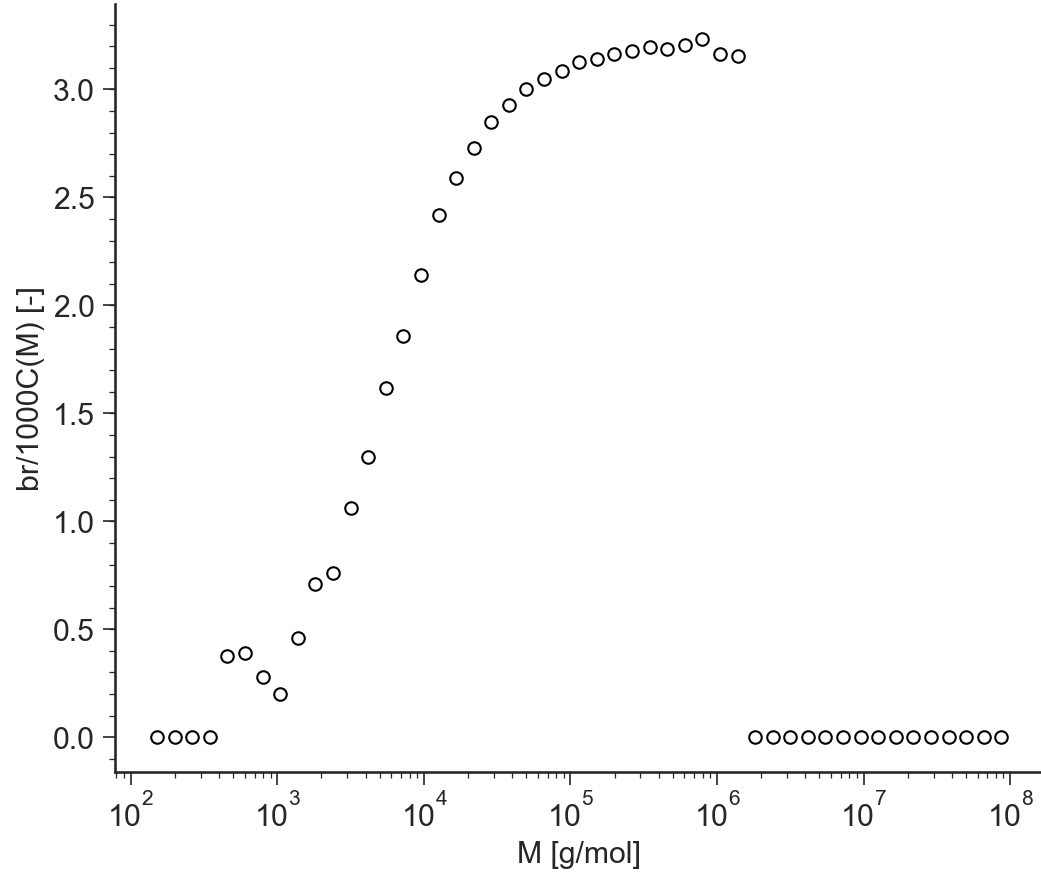# React: General description¶

## Purpose¶

Application for Monte Carlo polymerisation

## Data Files¶

• The first line of the file should contain the sample parameters separated by semi-colons (;). It may contain any number of parameters which will be read and saved as file-parameter in RepTate.

• Then the data columns should appear, separated by spaces or tabs.

### .reac extension¶

Text files with .reac extension should be organised as follows:

• .reac files are not expected to provide any specific parameter values.

• 4 columns separated by spaces or tabs containing respectively:

1. molar mass, $$M$$,

2. weight associated, $$\dfrac{\text d w(\log M)}{\text d \log M}$$,

3. the g-factor, $$g(M)=\dfrac{\langle R^2_g \rangle_\text{branched}}{\langle R^2_g \rangle_\text{linear}}$$

4. number of branching points per 1000 carbon.

A correct .reac file looks like:

0.1148E+03 0.5000E-03 0.1000E+01 0.0000E+00
0.1514E+03 0.1500E-02 0.1000E+01 0.0000E+00
0.1995E+03 0.1667E-02 0.1000E+01 0.0000E+00
0.2630E+03 0.1750E-02 0.1000E+01 0.0000E+00
0.3467E+03 0.5250E-02 0.1000E+01 0.0000E+00
0.4571E+03 0.6750E-02 0.9977E+00 0.3765E+00
...        ...        ...        ...


## Views¶

### w(M)¶

BaseApplicationReact.view_wM()[source]

Molecular weight distribution $$w(M)$$ vs molecular weight $$M$$ (in logarithmic scale)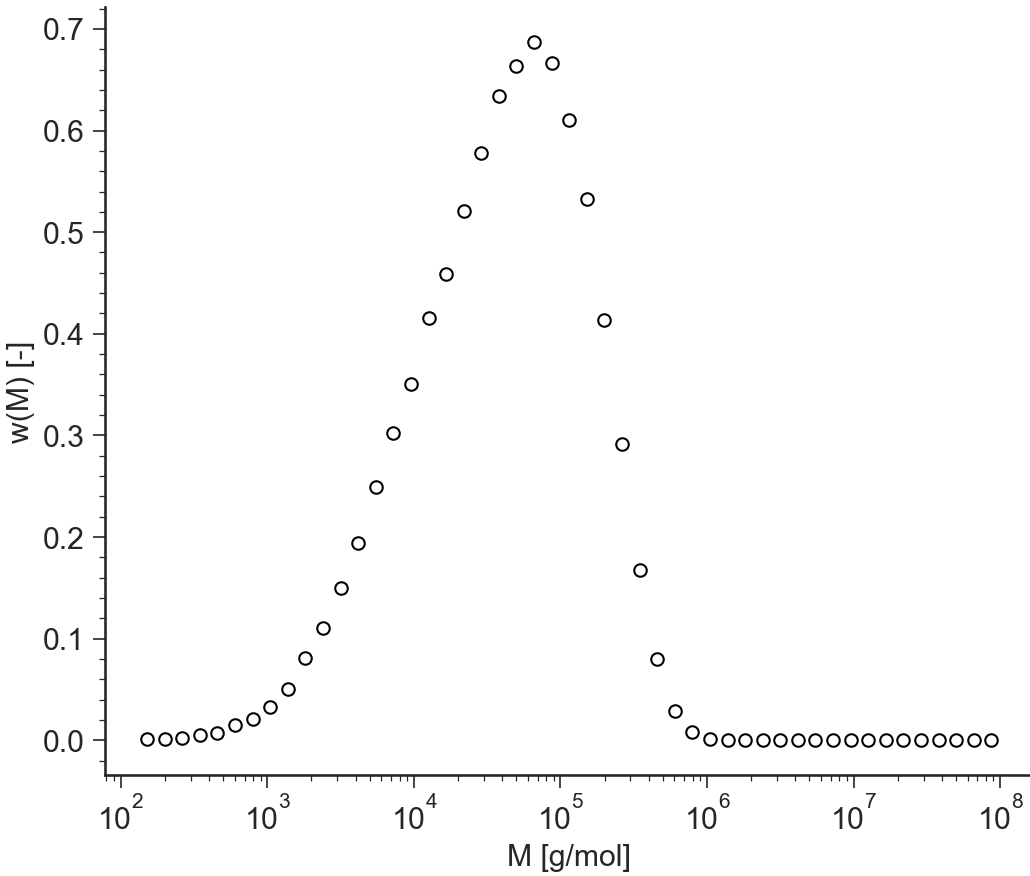### log(w(M))¶

$$\log_{10}(w(M))$$ (log-linear)

BaseApplicationReact.view_logwM()[source]

Logarithm of the molecular weight distribution $$\log(w(M))$$ vs molecular weight $$M$$ (in logarithmic scale)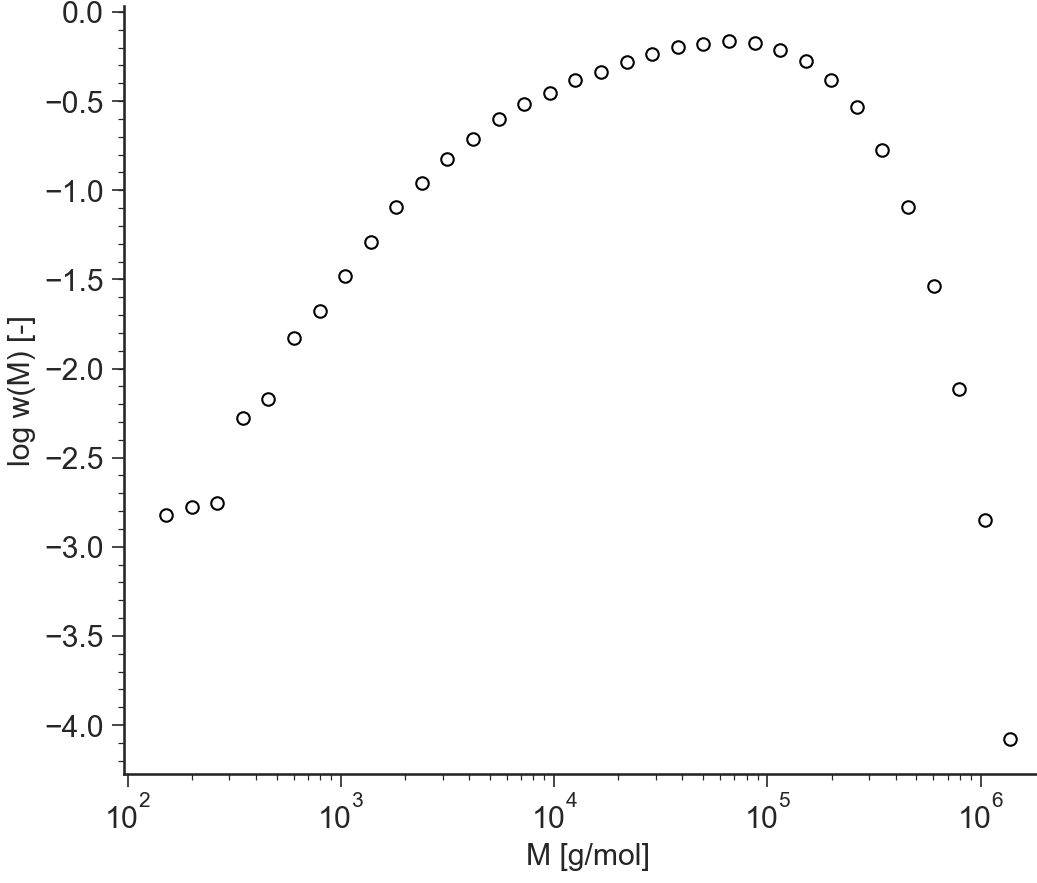### g(M)¶

$$g(M)$$ (log-linear)

BaseApplicationReact.view_gM()[source]

$$g$$-factor as a function of the molecular weight. The $$g$$-factor is defined as $$g = \dfrac{\langle R^2_g \rangle_\text{branched}}{\langle R^2_g \rangle_\text{linear}}$$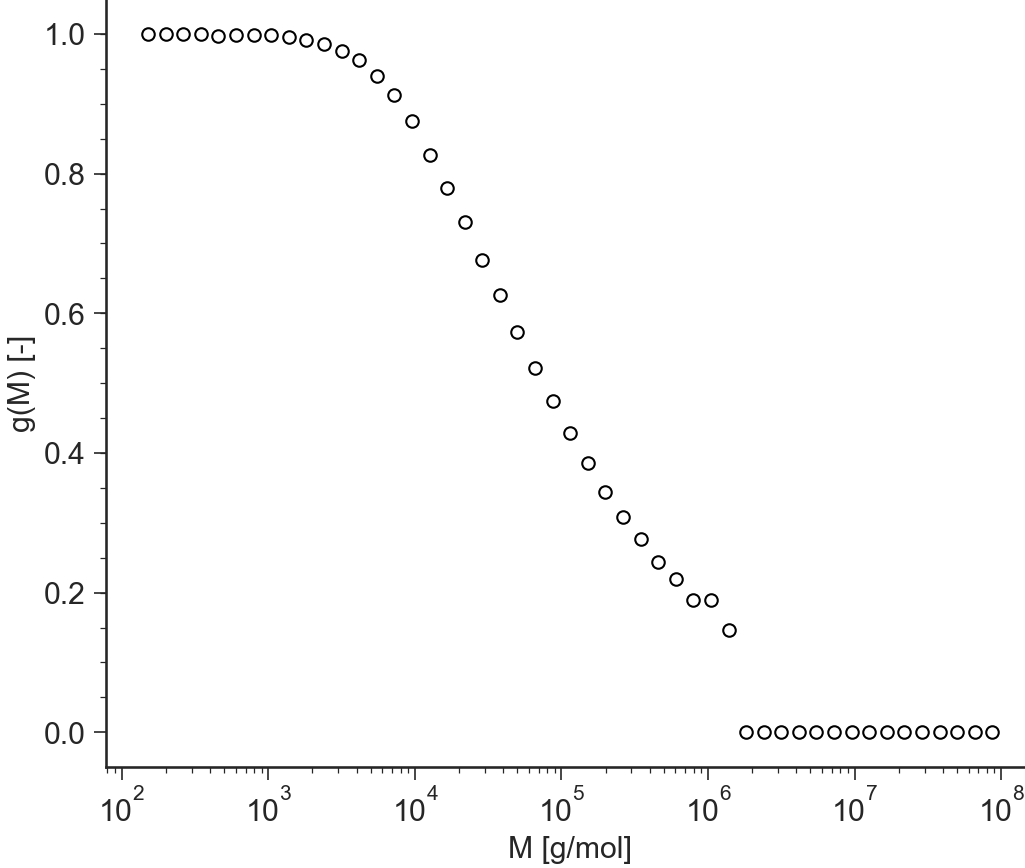### log(g(M))¶

$$\log_{10}(g(M))$$ (log-linear)

BaseApplicationReact.view_loggM()[source]

Logarithm of the $$g$$-factor as a function of the molecular weight. The $$g$$-factor is defined as $$g = \dfrac{\langle R^2_g \rangle_\text{branched}}{\langle R^2_g \rangle_\text{linear}}$$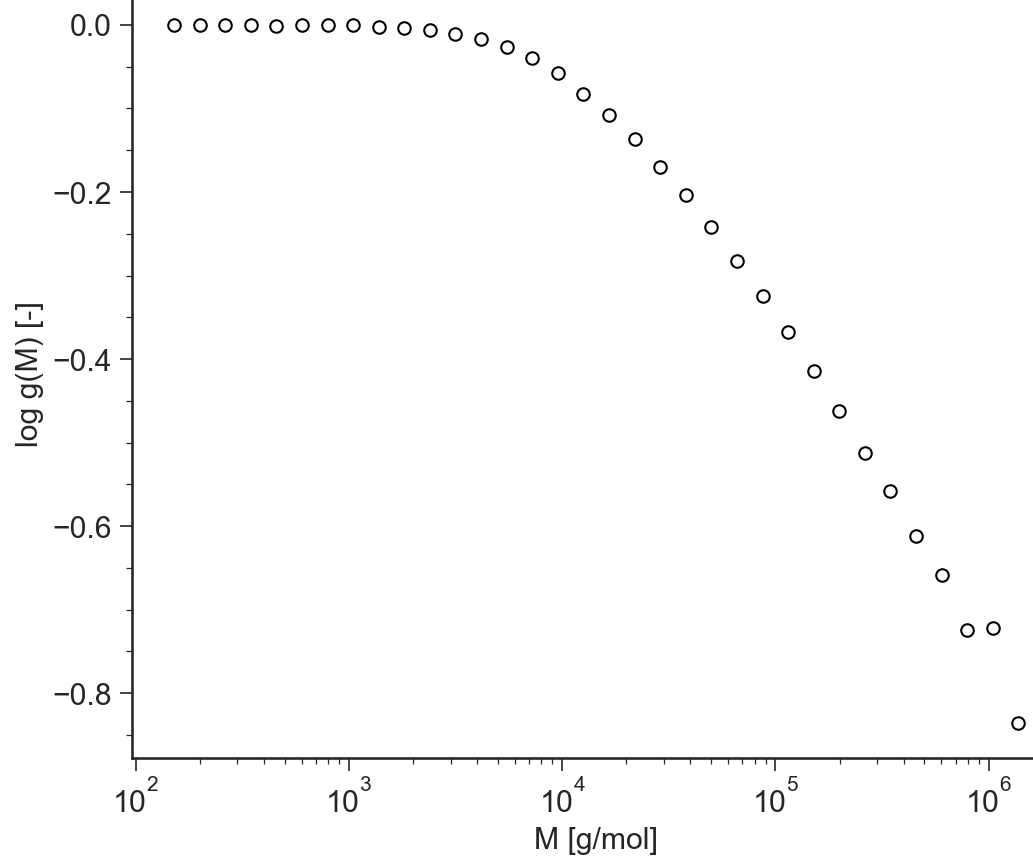### br/1000C¶

$$\text{br/1000C}$$ (log-linear)

BaseApplicationReact.view_br_1000C()[source]

Number of branching points per 1000 carbon as a function of the molecular weight